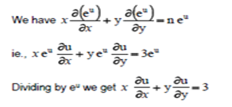Problems on Differential Calculus I-1: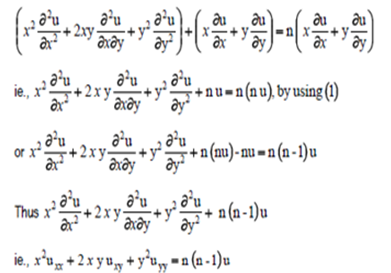9.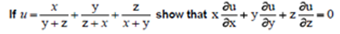Solution: Observe that the degree is 0 in every terms: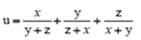We shall divide both numerator and denominator of every terms by x.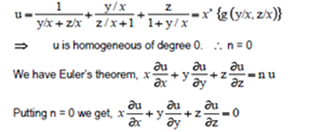10.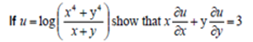Solution: We can put the given u in the form xn g (y/x).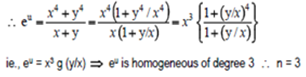Now applying Eulers Theorem for the homogenous functions: